Home > ACC7 > Chapter cc38 > Lesson cc38.2.1 > Problem8-55

8-55.
1. Simplify each expression. Homework Help ✎

1. 65

2.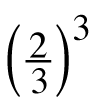3. (2 + 3)4

4.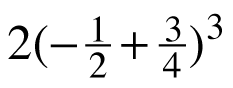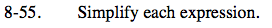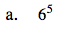6 · 6 · 6 · 6 · 6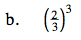$\frac{2}{3}\cdot\frac{2}{3}\cdot\frac{2}{3}$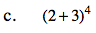Following the Order of Operations, first simplify the terms in parentheses.

(5)4

Then simplify the terms with exponents.

5 · 5 · 5 · 5

625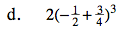Follow the Order of Operations.

$\frac{1}{32}$